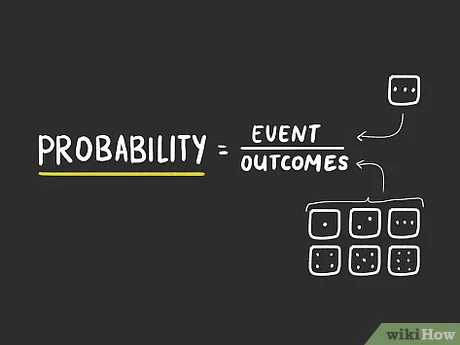Probability can be referred to as the branch of mathematics that measures the likelihood of an event or the occurrence of an event. Probability performs the role of analysis in decision making, it improves the decision making when faced with uncertainties, it helps decision making objectively and data-driven. Uncertainties can be said to be unknown or something that one is not sure about; for example, what is the probability that rainfall will improve the conditions of a farm product, this is uncertain and can only be solved with probability, analyzing the data.

Probability values are always 0-1, there are some statistical terms one has to be familiar with which will be defined below:

1. Experiment: can also be called trial, it is a procedure that has a set of possible outcomes
2. Sample space: can also be called sample description, it is the set of all possible outcomes or results of experiment.
3. Event: can be referred to as a set of outcomes of an experiment or a collection of sample points.
4. Complement: the probability that an event will not occur or the probability that 2 events both occur.
5. Intersection of events: the chances of two or more events occurring. It can be represented with AnB.
6. Union of events: is the set that contains the elements of 2 or more sets, it can be represented with AuB.

Assigning Probabilities

There are various approaches to assigning probability:

Classical method: is used if all the probable outcomes are given in advance and if all outcomes are equally likely.

Relative method: this focuses on the use of past or historical data and experimentation or the ratio of occurrence of a single event and the total number of events.

Subjective method: this method is derived or gotten from one’s personal judgment or experience about the occurrence of an event.

Relationships of probability

This has to do with the computation of events without knowledge of sample points:

Complement: the probability that an event will not occur or the probability that 2 events both occur. Complement of an event that contains all sample points not present in the event.

Union of events: is the set that contains the elements of 2 or more sets, it can be represented with AuB.

The intersection of events: the chances of two or more events occurring. It can be represented with AnB. It is the set of all sample points available in two events.

The addition law: provides a way of calculating the occurrence of multiple events. It is represented as P(AuB) = P(A) + P(B) – P(AnB)

Mutually exclusive: this explains that two events are exclusive if they have no sample points in common and if one event occurs and the other does not occur.

Conditional probability is the odds that an event will occur given that another event has occurred. It is represented with P(A|B) = P(AnB/ P(B)).## Next Generation Fixed Wireless Access (NGFWA) for Service Providers: A Viable Alternative to Traditional Fiber-Optic Networks

in## Transforming Nigeria’s Local Government System for Rural Economic Growth and Increased GDP

in